# km.surv

## Introduction

The km.surv function is part of the conf package. The function plots the probability mass function for the support values of Kaplan and Meier’s product–limit estimator1. The Kaplan-Meier product-limit estimator (KMPLE) is used to estimate the survivor function for a data set of positive values in the presence of right censoring. The km.surv function plots the probability mass function for the support values of the KMPLE for a particular sample size n, probability of observing a failure h at various the times of interest expressed as the cumulative probability associated with $$X = \min(T, C)$$, where $$T$$ is the failure time and $$C$$ is the censoring time under a random-censoring scheme.

## Installation Instructions

The km.surv function is accessible following installation of the conf package:

install.packages("conf")
library(conf)

## Details

The KMPLE is a nonparametric estimate of the survival function from a data set of lifetimes that includes right-censored observations and is used in a variety of application areas. For simplicity, we will refer to the object of interest generically as the item and the event of interest as the failure.

Let $$n$$ denote the number of items on test.The KMPLE of the survival function $$S(t)$$ is given by $\hat{S}(t) = \prod\limits_{i:t_i \leq t}\left( 1 - \frac{d_i}{n_i}\right),$ for $$t \ge 0$$, where $$t_1, \, t_2, \, \ldots, \, t_k$$ are the times when at least one failure is observed ($$k$$ is an integer between 1 and $$n$$, which is the number of distinct failure times in the data set), $$d_1, \, d_2, \, \ldots, \, d_k$$ are the number of failures observed at times $$t_1, \, t_2, \, \ldots, \, t_k$$, and $$n_1, \, n_2, \, \ldots, \, n_k$$ are the number of items at risk just prior to times $$t_1, \, t_2, \, \ldots, \, t_k$$. It is common practice to have the KMPLE “cut off” after the largest time recorded if it corresponds to a right-censored observation2. The KMPLE drops to zero after the largest time recorded if it is a failure; the KMPLE is undefined (NA), however, after the largest time recorded if it is a right-censored observation.

The support values, S, are calculated in km.support from $$\hat{S}(t)$$ at any $$t \ge 0$$ for all possible outcomes of an experiment with $$n$$ items on test. These values, along with NA, are on the $$y$$-axis of the plot produced by km.surv.

The probabilities of each support value are calculated using the km.pmf function from the conf package. This function also calculates the probability of NA, the event that the last time recorded is a right-censored observation. These probabilities are plotted through the function km.surv. The probabilities are reflected by different sizes of the dots in the plot. As an alternative to using area to indicate the relative probability, km.surv can plot the probability mass functions using grayscales (by setting graydots = TRUE). One of the two approaches might work better in different scenarios.

In addition, when ev is set to TRUE, the expected values are plotted in red. They are calculated by removing the probability of NA and normalizing over the rest of the probabilities.

### Required Arguments

n sample size

h probability of observing a failure; that is, P(X = T)

### Optional Arguments

lambda plotting frequency of the probability mass functions (default is 10)

ev option to plot the expected values of the support values (default is FALSE)

line option to connect the expected values with lines (default is FALSE)

graydots option to express the weight of the support values using grayscale (default is FALSE)

gray.cex option to change the size of the gray dots (default is 1)

gray.outline option to display outlines of the gray dots (default is TRUE)

xfrac option to label support values on the y-axis as exact fractions (default is TRUE)

## Examples

The following section provides various examples for the usage of km.surv.

### Example 1

Qin et al.3 derived the probability mass function of the KMPLE for one particular setting where there are n = 3 items on test, the failure times $$T_1,T_2$$ and $$T_3$$ and the censoring times $$C_1,C_2$$ and $$C_3$$ both follow an exponential(1) distribution. The fixed time of interest is $$t_0 = -\ln(1/2)/2$$, which is the median of $$X = \min(T, C)$$, where $$T$$ is the failure time and $$C$$ is the censoring time under a random-censoring scheme. Therefore, perc = 0.5.

In this case, since failure and censoring times have the same exponential distribution, they are equally likely to occur; that is, h = 1/2.

For this example, km.surv is called with the arguments n = 3, h = 1/2. To compare this with Example 1 in the km.pmf vignette, look at the plot where the cumulative probability of X = 0.5 on the $$x$$-axis. Since the default of lambda = 10, the times of interest are 0 to 1 at every 10th percentile.

library(conf)
#  display the probability mass functions at various times of interest

km.surv(n = 3, h = 1/2)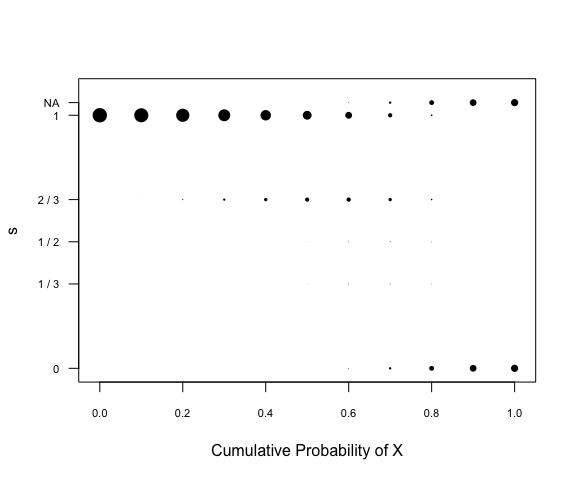### Example 2

A more interesting example is with n = 4 and two probabilities of failure. For the first plot set a probability of failure h = 1/3. Increasing lambda to 100 and including the expected values connected by red lines produces a very interesting plot. The probability mass functions have larger probabilities of 1 due to the higher rate of censoring. The KMPLE remains at 1 until the first failure so all possible censored items that come before that first failure is considered in this probability. The high probability of right-censored items is also evident at the end of the experiment when there is a high probability that the last item is censored resulting in a high probability that there will be an NA.

#  display the probability mass function at various times of interest
#  with the expected values in red connected with lines

km.surv(n = 4, h = 1/3, lambda = 100, ev = TRUE, line = TRUE)
title("High Censoring Rate")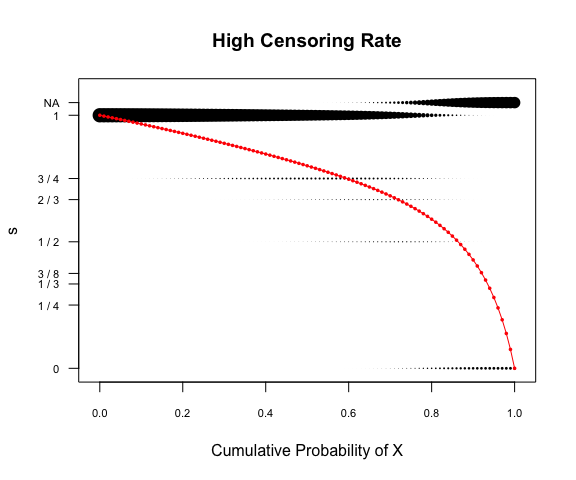In contrast with the high probability of right-censoring, the high probability of a failure h = 2/3 results in the following plot. We see an initial high probability of 1 that decays quicker since there is less chance of there being censored items before the first failure and a low probability of NA at the end of the experiment since there is a higher probability that the last item will fail over being censored.

#  display the probability mass function at various times of interest
#  with the expected values in red connected with lines

km.surv(n = 4, h = 2/3, lambda = 100, ev = TRUE, line = TRUE)
title("High Failure Rate")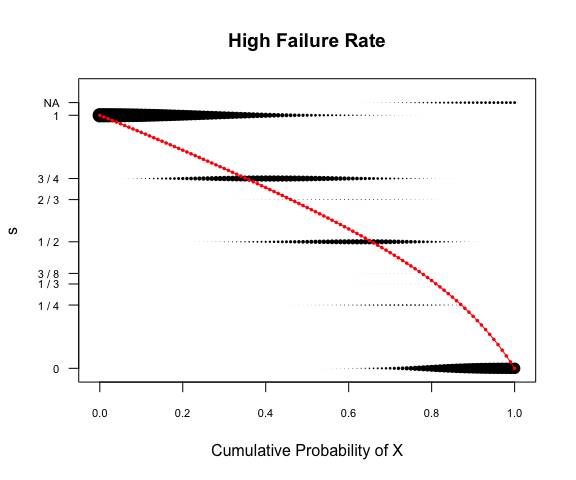### Example 3

The function km.surv provides many arguments to make the plot as useful as possible. For example, when n is larger, the plot may be improved by using decimals instead of the exact fractions (xfrac = FALSE) or gray dots where the intensity is related to the probability instead of the size (graydots = TRUE). When probabilities are too small to be seen, gray outlines circle them. This option can be turned off with gray.outline = FALSE. The size of the dots can be made smaller or larger using gray.cex where the default is 1.

#  display the probability mass function at various times of interest
km.surv(n = 7, h = 3/4, lambda = 50, graydots = TRUE, xfrac = FALSE)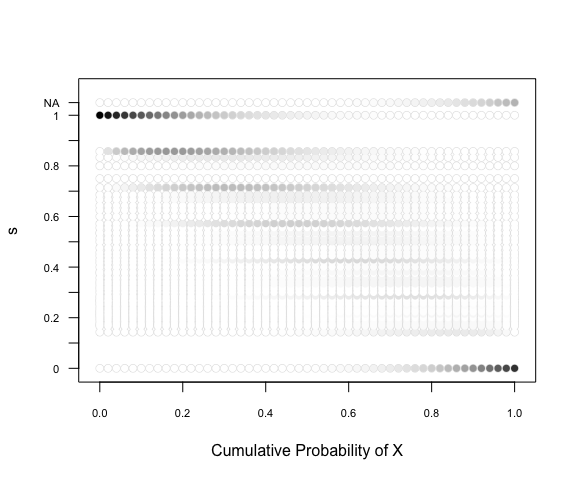Removing the outlines that accentuates the small probabilities produces a less busy plot.

#  display the probability mass function at various times of interest
km.surv(n = 7, h = 3/4, lambda = 50, graydots = TRUE, xfrac = FALSE, gray.outline = FALSE)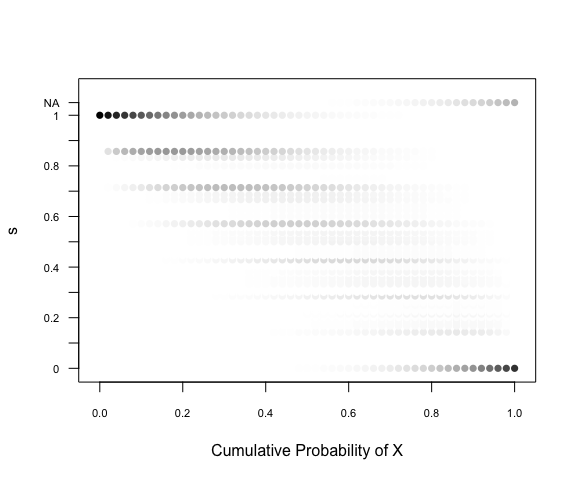Removing the outlines, increasing the dot size, and adding expected values to a plot with sample size of 5 and a slighter higher rate of failure than censoring, produces the following plot.

#  display the probability mass function at various times of interest
km.surv(n = 5, h = 5/8, lambda = 30, graydots = TRUE, ev = TRUE, gray.outline = FALSE, gray.cex = 1.25)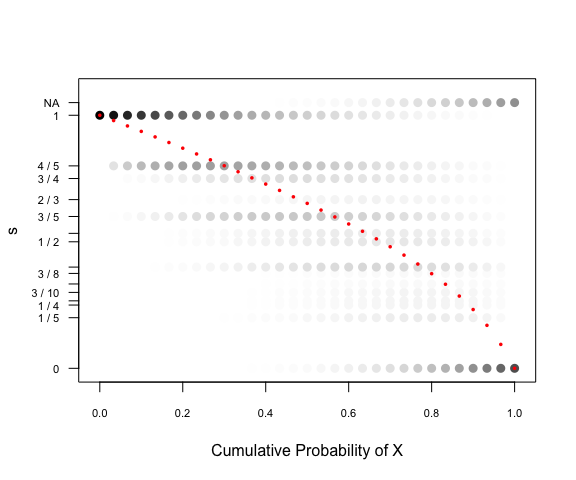## Package Notes

For more information on how the $$\hat{S}(t)$$ values are generated, please refer to the vignette titled km.support.

For more information on calculation of the probabilities of the support values, please refer to the vignette titled km.pmf.

In addition, km.surv calls the functions km.support and km.pmf.

These functions and vignettes are both available via the link on the conf package webpage.

1. Kaplan, E. L., and Meier, P. (1958), “Nonparametric Estimation from Incomplete Observations,” Journal of the American Statistical Association, 53, 457–481.↩︎

2. Kalbfleisch, J. D., and Prentice, R. L. (2002), The Statistical Analysis of Failure Time Data (2nd ed.), Hoboken, NJ: Wiley.↩︎

3. Qin Y., Sasinowska H. D., Leemis L. M. (2023), “The Probability Mass Function of the Kaplan–Meier Product–Limit Estimator,” The American Statistician, 77 (1), 102–110.↩︎